# GCSE Maths Paper 06 (Higher) Practice Paper in the Style of OCR - Calculator

In this assessment, students will be able to complete a timed GCSE Mathematics Paper 06 (Calculator) in the style of OCR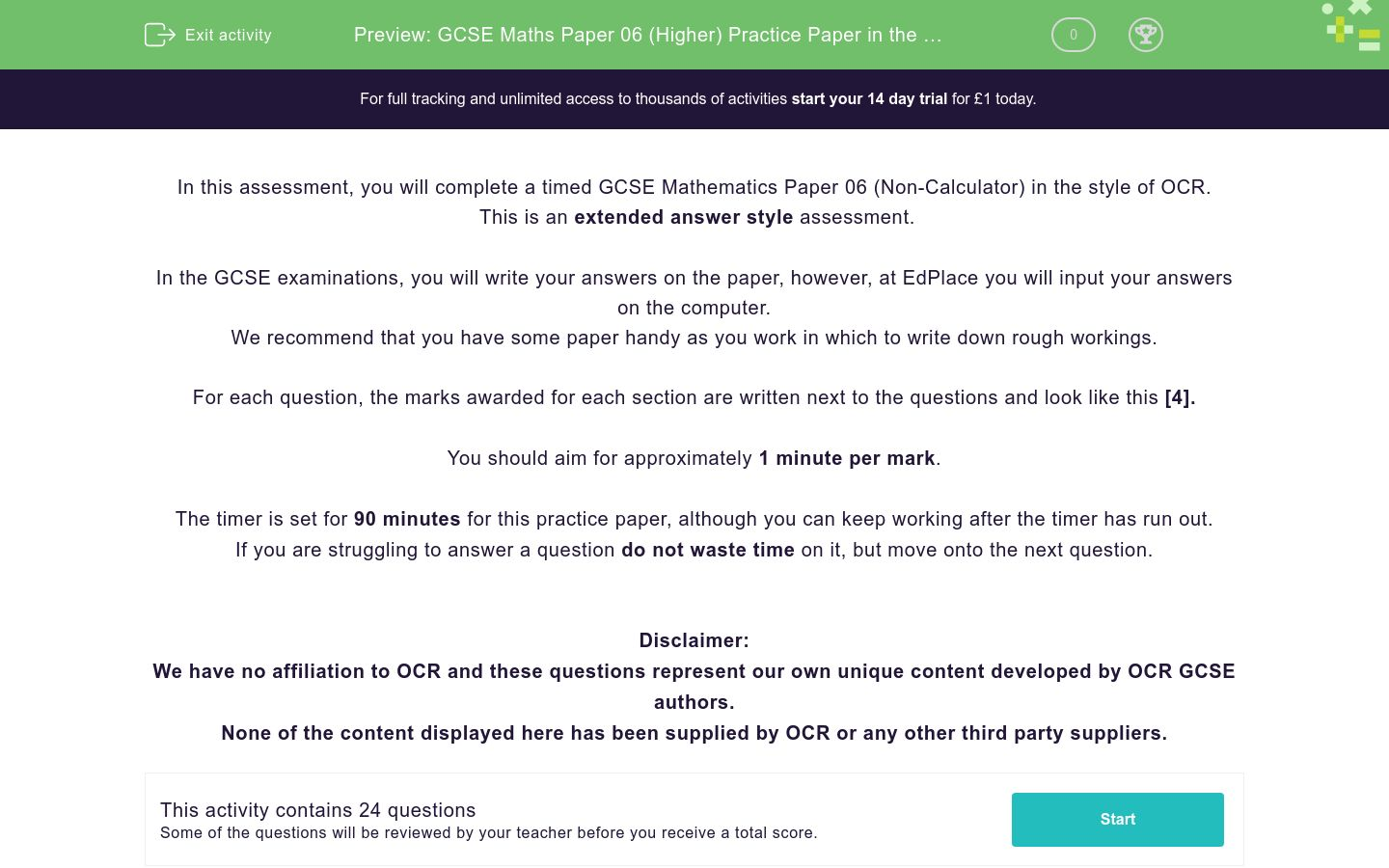Key stage:  KS 4

GCSE Subjects:   Maths

GCSE Boards:   OCR

Curriculum topic:   Higher Practice Papers

Curriculum subtopic:   Calculator Practice Papers

Difficulty level:### QUESTION 1 of 10

In this assessment, you will complete a timed GCSE Mathematics Paper 06 (Non-Calculator) in the style of OCR.

This is an extended answer style assessment.

In the GCSE examinations, you will write your answers on the paper, however, at EdPlace you will input your answers on the computer.

We recommend that you have some paper handy as you work in which to write down rough workings.

For each question, the marks awarded for each section are written next to the questions and look like this .

You should aim for approximately 1 minute per mark.

The timer is set for 90 minutes for this practice paper, although you can keep working after the timer has run out.

If you are struggling to answer a question do not waste time on it, but move onto the next question.

Disclaimer:

We have no affiliation to OCR and these questions represent our own unique content developed by OCR GCSE authors.

None of the content displayed here has been supplied by OCR or any other third party suppliers.

ξ = {1,2,3,4,5,6,7,8,9,10,11,12,13,14,15,16,17,18,19,20}

A = {Multiples of 3}

B = {Factors of 18}

(a) Complete the Venn diagram to represent this informationA number is chosen at random from the universal set ξ

(b) What is the probability the number is in the set AUB?                                

Solve the simultaneous equations

3x + 2y = 9

4x – 3y = 3.5



(a) Complete this table of values for y = x2 + 2x – 1               

 x -4 -3 -2 -1 0 1 2 y 7 -1 2

(b) Draw the graph of y = x2 + 2x – 1 for -4 ≤ x ≤ 2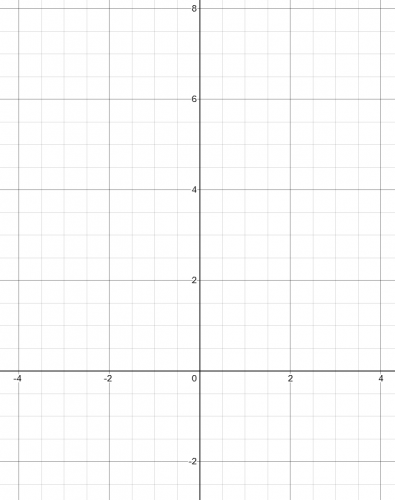(c) Use the graph to estimate a solution to x2 +2x – 1 = 0          

ABC is a right-angled triangle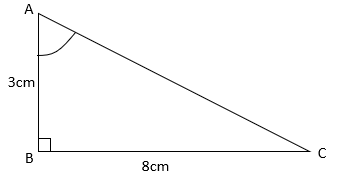(a) Work out the size of angle CAB.                                

The length of side BC is increased by 2 cm

The length of AB stays the same

Triangle ABC is still right angled.

(b) Will the value of tan CAB increase or decrease? Give a reason for your answer.                                

In the diagram A,B and C are three regular polygons.David thinks that a square will fit into the space for polygon X.

Is he correct?



Doris wants to paint a gas tank sat in her garden.

The gas tank is in the shape of a cylinder and she wants to paint all the sides including the top and bottom.The gas tank has a diameter of 2.2 metres and a height of 1.3 metres.

Doris has 6 tins of paint that each cover 3.2 m2.

Does she have enough paint to cover the tank?



SolveA metal cube has a total surface area of 54cm2.

It is melted down and used to make spheres with a radius of 9mm.

What is the maximum number of spheres that could be made?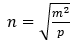m = 3.2 x 105

p = 4.3 x 104

(a) Find the value of n.

Give your answer in standard form correct to 3 significant figures   

m is decreased by 5%

p is decreased by 15%

John says ‘n must be smaller because both m and p are smaller’

(b) Is John correct?

Three toy cars go around a track.

Car A completes a circuit every 40 seconds

Car B completes a circuit every 65 seconds

Car C completes a circuit every 70 seconds.

All three cars start at the same time from the start line.

Will all three cars cross the start line together within one hour?



Here is a pyramid with a square base ABCD.AB = 6 cm

The vertex T is 8 cm above the midpoint of BD.

Calculate the size of angle ATC.



Here are two similar tins of paintThe volume of tin A: the volume of tin B = 4:3

The surface area of the label on tin B is 100 cm2

Work out the surface area of the label on tin A.



Simplify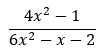Rearrange the equation to make x the subject.The graph shows the speed of a coach, in metres per second during the first 20 seconds of a journey.(a) Use the graph to estimate of the distance travelled in the first 20 seconds.

Use 5 strips of equal width.                       

(b) Is your answer to part (a) an underestimate or an overestimate of the actual distance travelled by the coach in the first 20 seconds?The area of this triangle is (10√3) m2

Calculate the value of X, giving your answer to 3 significant figures



Solve the inequality.

2x2 – 3x – 9 < 0



(a) Show that the equation x3 + 2x = 2 has a solution between 0 and 1.                 

(b) Show that the equation x3 + 2x = 2 can be rearranged to give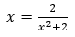(c) Starting with x0 = 0.5,

Use the iteration formula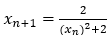three times to find an estimate for the solution of x3 + 2x = 2                

Solve algebraically the simultaneous equations.

x2 + 3y2 = 10

2x + 3y = 7



Here are the first five terms of a quadratic sequence, the nth term of this quadratic sequence is an2 + bn + c

10    21    38   61    90

Find the values of a, b and c.



Solve by factorisation.

6x2 – 5x – 6 = 0



BCD is a parallelogram.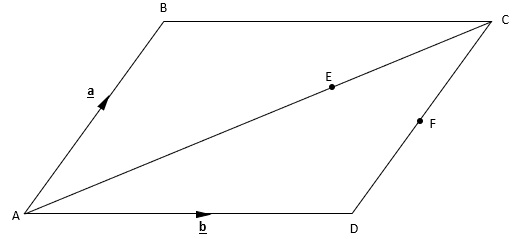AB = a and AD = b

E is the point that splits AC into the ratio 2:1

F is the midpoint of CD

a) Write down simplified expressions in terms of a and b for…

i) AC                                   

ii) AE                                    

b) Show that BE = 1/3 (-a + 2b)                    

c) Prove that the points b, e and f are on a straight line.                

a) Write x2 – 6x – 7 in the form (x – a)2 – b                     

b) Describe the single transformation which maps the graph of y = x2 onto the graph for y = x2 – 6x – 7                  

ABD and BCD are triangles.a) Show that BD = 11.8 cm, correct to 3 significant figures.                 

• Question 1

ξ = {1,2,3,4,5,6,7,8,9,10,11,12,13,14,15,16,17,18,19,20}

A = {Multiples of 3}

B = {Factors of 18}

(a) Complete the Venn diagram to represent this informationA number is chosen at random from the universal set ξ

(b) What is the probability the number is in the set AUB?                                

EDDIE SAYS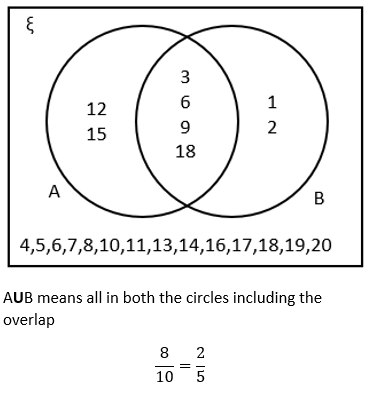• Question 2

Solve the simultaneous equations

3x + 2y = 9

4x – 3y = 3.5



EDDIE SAYS• Question 3

(a) Complete this table of values for y = x2 + 2x – 1               

 x -4 -3 -2 -1 0 1 2 y 7 -1 2

(b) Draw the graph of y = x2 + 2x – 1 for -4 ≤ x ≤ 2(c) Use the graph to estimate a solution to x2 +2x – 1 = 0          

EDDIE SAYS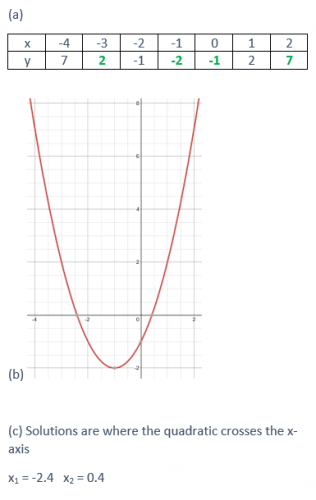• Question 4

ABC is a right-angled triangle(a) Work out the size of angle CAB.                                

The length of side BC is increased by 2 cm

The length of AB stays the same

Triangle ABC is still right angled.

(b) Will the value of tan CAB increase or decrease? Give a reason for your answer.                                

EDDIE SAYS• Question 5

In the diagram A,B and C are three regular polygons.David thinks that a square will fit into the space for polygon X.

Is he correct?



EDDIE SAYS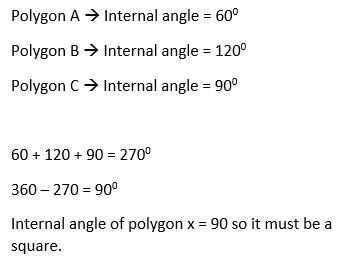• Question 6

Doris wants to paint a gas tank sat in her garden.

The gas tank is in the shape of a cylinder and she wants to paint all the sides including the top and bottom.The gas tank has a diameter of 2.2 metres and a height of 1.3 metres.

Doris has 6 tins of paint that each cover 3.2 m2.

Does she have enough paint to cover the tank?



EDDIE SAYS• Question 7

SolveEDDIE SAYS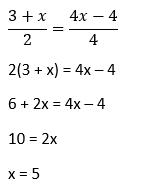• Question 8

A metal cube has a total surface area of 54cm2.

It is melted down and used to make spheres with a radius of 9mm.

What is the maximum number of spheres that could be made?EDDIE SAYS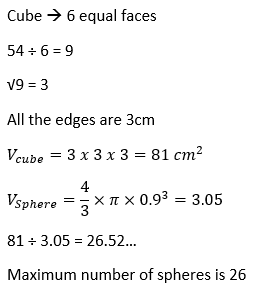• Question 9m = 3.2 x 105

p = 4.3 x 104

(a) Find the value of n.

Give your answer in standard form correct to 3 significant figures   

m is decreased by 5%

p is decreased by 15%

John says ‘n must be smaller because both m and p are smaller’

(b) Is John correct?

EDDIE SAYS• Question 10

Three toy cars go around a track.

Car A completes a circuit every 40 seconds

Car B completes a circuit every 65 seconds

Car C completes a circuit every 70 seconds.

All three cars start at the same time from the start line.

Will all three cars cross the start line together within one hour?



EDDIE SAYS• Question 11

Here is a pyramid with a square base ABCD.AB = 6 cm

The vertex T is 8 cm above the midpoint of BD.

Calculate the size of angle ATC.



EDDIE SAYS• Question 12

Here are two similar tins of paintThe volume of tin A: the volume of tin B = 4:3

The surface area of the label on tin B is 100 cm2

Work out the surface area of the label on tin A.



EDDIE SAYS• Question 13

SimplifyEDDIE SAYS• Question 14

Rearrange the equation to make x the subject.EDDIE SAYS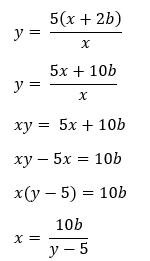• Question 15

The graph shows the speed of a coach, in metres per second during the first 20 seconds of a journey.(a) Use the graph to estimate of the distance travelled in the first 20 seconds.

Use 5 strips of equal width.                       

(b) Is your answer to part (a) an underestimate or an overestimate of the actual distance travelled by the coach in the first 20 seconds?

EDDIE SAYS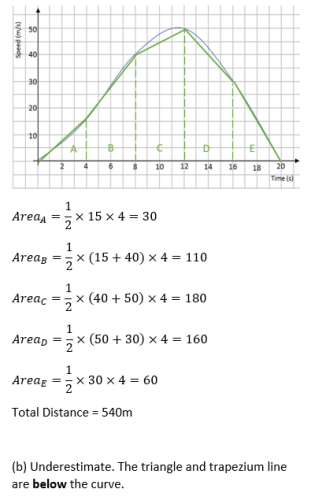• Question 16The area of this triangle is (10√3) m2

Calculate the value of X, giving your answer to 3 significant figures



EDDIE SAYS• Question 17

Solve the inequality.

2x2 – 3x – 9 < 0



EDDIE SAYS• Question 18

(a) Show that the equation x3 + 2x = 2 has a solution between 0 and 1.                 

(b) Show that the equation x3 + 2x = 2 can be rearranged to give(c) Starting with x0 = 0.5,

Use the iteration formulathree times to find an estimate for the solution of x3 + 2x = 2                

EDDIE SAYS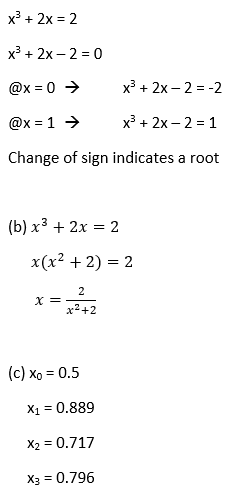• Question 19

Solve algebraically the simultaneous equations.

x2 + 3y2 = 10

2x + 3y = 7



EDDIE SAYS• Question 20

Here are the first five terms of a quadratic sequence, the nth term of this quadratic sequence is an2 + bn + c

10    21    38   61    90

Find the values of a, b and c.



EDDIE SAYS• Question 21

Solve by factorisation.

6x2 – 5x – 6 = 0



EDDIE SAYS• Question 22

BCD is a parallelogram.AB = a and AD = b

E is the point that splits AC into the ratio 2:1

F is the midpoint of CD

a) Write down simplified expressions in terms of a and b for…

i) AC                                   

ii) AE                                    

b) Show that BE = 1/3 (-a + 2b)                    

c) Prove that the points b, e and f are on a straight line.                

EDDIE SAYS• Question 23

a) Write x2 – 6x – 7 in the form (x – a)2 – b                     

b) Describe the single transformation which maps the graph of y = x2 onto the graph for y = x2 – 6x – 7                  

EDDIE SAYS• Question 24

ABD and BCD are triangles.a) Show that BD = 11.8 cm, correct to 3 significant figures.                 

EDDIE SAYSTry it ---- OR ----

Sign up for a £1 trial so you can track and measure your child's progress on this activity.

### What is EdPlace?

We're your National Curriculum aligned online education content provider helping each child succeed in English, maths and science from year 1 to GCSE. With an EdPlace account you’ll be able to track and measure progress, helping each child achieve their best. We build confidence and attainment by personalising each child’s learning at a level that suits them.

Get started